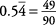## Changing Infinite Repeating Decimals to Fractions

Remember: Infinite repeating decimals are usually represented by putting a line over (sometimes under) the shortest block of repeating decimals. Every infinite repeating decimal can be expressed as a fraction.

Find the fraction represented by the repeating decimal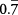.

Let n stand for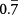or 0.77777 …

So 10 n stands foror 7.77777 …

10 n and n have the same fractional part, so their difference is an integer.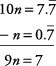You can solve this problem as follows.So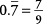Find the fraction represented by the repeating decimal.

Let n stand foror 0.363636 …

So 10 n stands for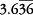or 3.63636 …

and 100 n stands for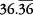or 36.3636 …

100 n and n have the same fractional part, so their difference is an integer. (The repeating parts are the same, so they subtract out.)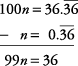You can solve this equation as follows:Now simplifyto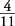.

SoFind the fraction represented by the repeating decimal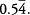.

Let n stand for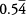or 0.544444 …

So 10 n stands for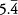or 5.444444 …

and 100 n stands for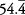or 54.4444 …

Since 100 n and 10 n have the same fractional part, their difference is an integer. (Again, notice how the repeated parts must align to subtract out.)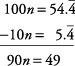You can solve this equation as follows.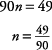So